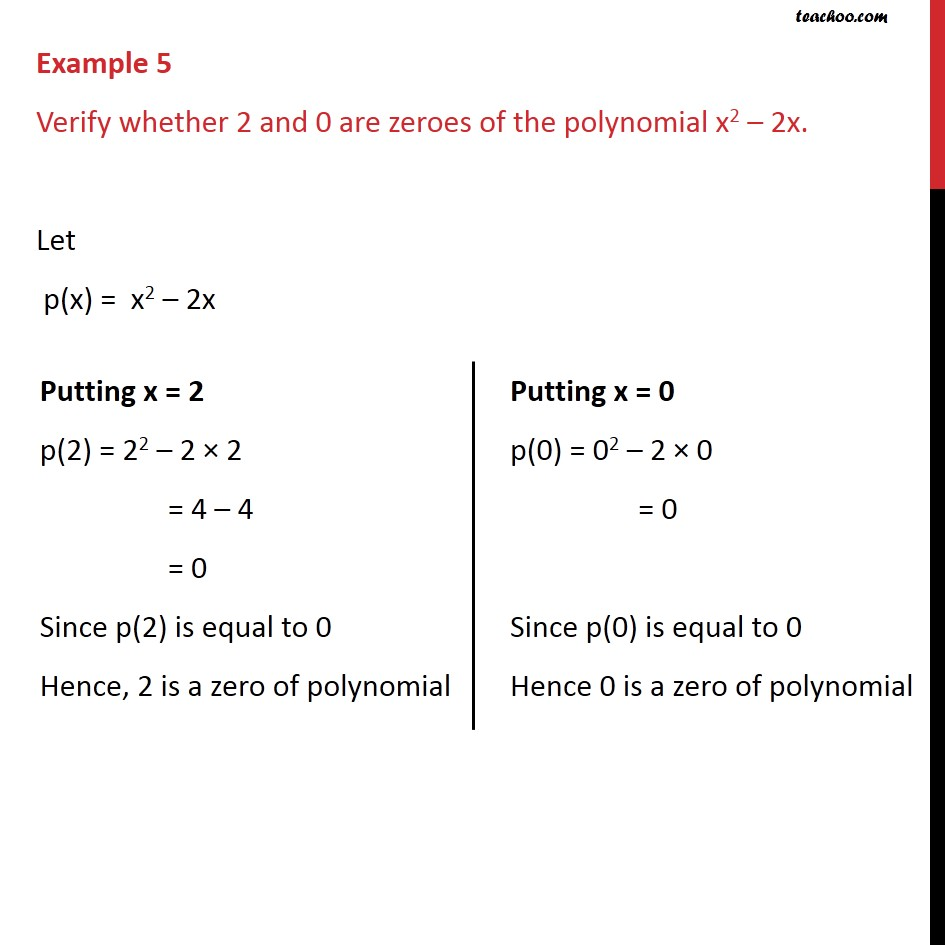1. Chapter 2 Class 9 Polynomials (Term 2)
2. Concept wise
3. Verifying Zeroes of a polynomial

Transcript

Example 5 Verify whether 2 and 0 are zeroes of the polynomial x2 2x. Let p(x) = x2 2x Putting x = 2 p(2) = 22 2 2 = 4 4 = 0 Since p(2) is equal to 0 Hence, 2 is a zero of polynomial Putting x = 0 p(0) = 02 2 0 = 0 Since p(0) is equal to 0 Hence 0 is a zero of polynomial